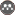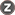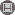Volume 5, Issue 2 (Summer 2018)                   johe 2018, 5(2): 1-11 | Back to browse issues page

BibTeX | RIS | EndNote | Medlars | ProCite | Reference Manager | RefWorks
Send citation to:oliaei M, Golmohammadi R, Aliabadi M, Shahidi R, Orvati movafagh M. Comparison of Static Wave Ratio and transfer Function Method in Determining the Sound Absorption Coefficient of Materials. johe. 2018; 5 (2) :1-11
URL: http://johe.umsha.ac.ir/article-1-361-en.html
Hamadan University of Medical Sciences , m.oliaei@edu.umsha.ac.ir
Abstract:   (1107 Views)
Introduction: Sound absorption coefficient determination is one of the most important factors among material selection for indoor noise control. The objectives of this study were: 1) comparison sound absorption coefficient of different materials by standing wave ratio and transfer function methods, and 2) developing a regression model in adjusting provided results.
Methods: In this study, 46 acoustic materials were selected and tested. In order to measure the absorption coefficient of materials two instrument called the impedance tube model 9410 made in the Iranian company AvaSina, and the impedance tube BSWA model SW 260 in 125 to 2000 Hz frequency range were utilized.
Results: The results obtained from the regression model shown that frequency of 500 Hz has the highest correlation (R=0.968, R2=0.936), and the lowest correlation coefficient was founded at 125 Hz (R=0.368, R2=0.136). Also, the value 0.829 was obtained for correlation coefficient of NRC.
Conclusions: The results showed that there were good agreement at 250, 500, 1000 and 2000 Hz frequencies between two methods. It can be concluded that the standing wave ratio method is a reliable approach in determining sound absorption coefficient of acoustic materials.